output.to from Sideway
Mechanics: Statics

1

Draft for Information Only

# Force Couple

A force couple is a system of forces that exerts only a resultant moment MO on an object with zero resultant force. The simplest force couple is two parallel forces, F1 and F2 along two lines of action at a distance apart with same magnitude F but opposite in sense,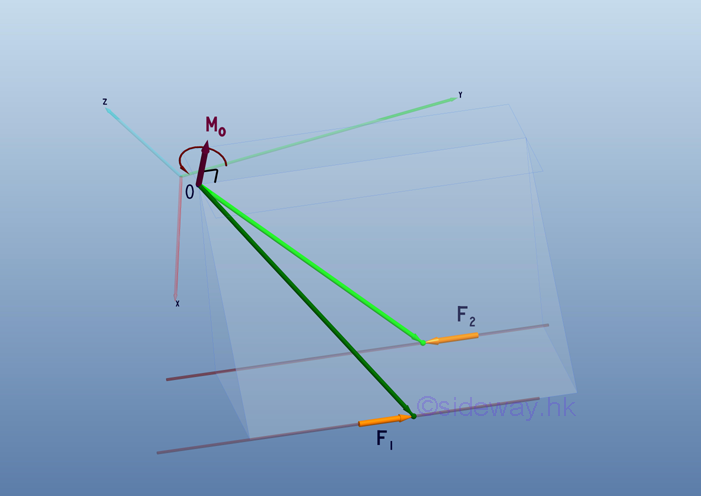Moment of a couple

Moment of a couple is the effective moment of a pair of equal and opposite coplanar forces.The resultant moment of forces, F1 and F2 about a point O can be expressed as: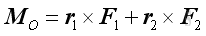Physically, the moments due to the two parallel forces, F1 and F2  are always lying on a plane normal to the forces, F1 and F2.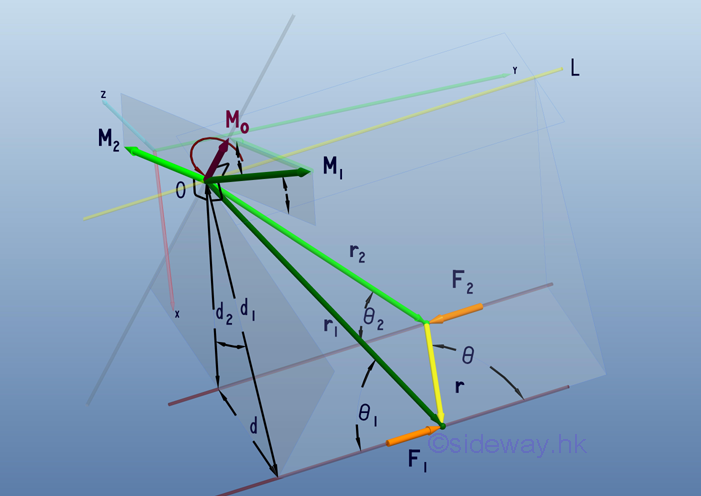The angle between the prependicular moment arms, d1 and d2 is always the supplementary angle of the angle between moment vectors, M1 and M2. Since the magnitudes of forces F1 and F2  are the same, the magnitudes of moments M1 and M2 are proportional to the prependicular moment arm only. Therefore the triangle formed by moments,  M1, M2 and MO  and the triangle formed by distances  d1 d2 and d  are similar triangles. Magnitude of the result moment MO is alway equal to force F times distance d. By the law of cosine, imply: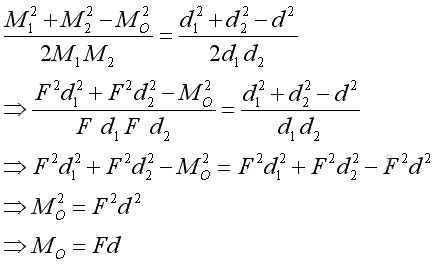The magnitude of the resultant moment  MO  is independent from the reference point. Moment M1 is always normal to prependicular moment arms, d1. It implys that moment  MO  is alway normal to the plane of the coplanar forces, F1 and F2.

Geometrically, the intersection of planes passing through the reference point O and normal to M1 and M2 also forms a line OL through the reference point O parallel to the forces, F1 and F2. The resultant moment about any reference point along line OL will be the same because both force and moment arm does not change along line OL.

Therefore the resultant moment  MO  is a free vector independent from the reference point. The direction of the resultant moment  MO  is  alway normal to the coplanar forces , F1 and F2 . The magnitude of the  resultant moment  MO  is  alway equals to the magnitude of the force couple, F time the prependicular distance of the two parallel lines of action apart, d.

Moment vector of a couple

The resultant moment MO about a reference point is the sum of the moments of the two forces of the couple. Since the two forces of a couple are equal in magnitude and opposite in sense. According to the right hand rule, ImplyTherefore the resultant moment vector MO is normal to the plane containing the coplanar force couple and the magnitude of the resultant moment vector  MO is: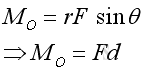Since the resultant moment vector MO is a cross product of moment arm r and the positive force vector F, it is independent of the choice of the reference point.

©sidewayReferences

1. I.C. Jong; B.G. rogers, 1991, Engineering Mechanics: Statics and Dynamics, Saunders College Publishing, United States of America
2. F.P. Beer; E.R. Johnston,Jr.; E.R. Eisenberg, 2004, Vector Mechanics for Engineers: Statics, McGraw-Hill Companies, Inc., New YorkID: 110600005 Last Updated: 6/22/2011 Revision: 0 Ref:Home (5)

Business

Management

HBR (3)

Information

Recreation

Hobbies (7)

Culture

Chinese (1097)

English (336)

Reference (66)

Computer

Hardware (149)

Software

Application (187)

Digitization (24)

Numeric (19)

Programming

Web (644)CSS (SC)

ASP.NET (SC)

HTML

Knowledge Base

Common Color (SC)

Html 401 Special (SC)

OS (389)MS Windows

Windows10 (SC)

.NET Framework (SC)

DeskTop (7)

Knowledge

Mathematics

Formulas (8)

Number Theory (206)

Algebra (20)

Trigonometry (18)

Geometry (18)

Calculus (67)

Complex Analysis (21)

Engineering

Tables (8)

Mechanical

Mechanics (1)

Rigid Bodies

Statics (92)

Dynamics (37)

Fluid (5)

Control

Acoustics (19)

Biology (1)

Geography (1)

Latest Updated Links

Copyright © 2000-2019 Sideway . All rights reserved Disclaimers last modified on 10 Feb 2019